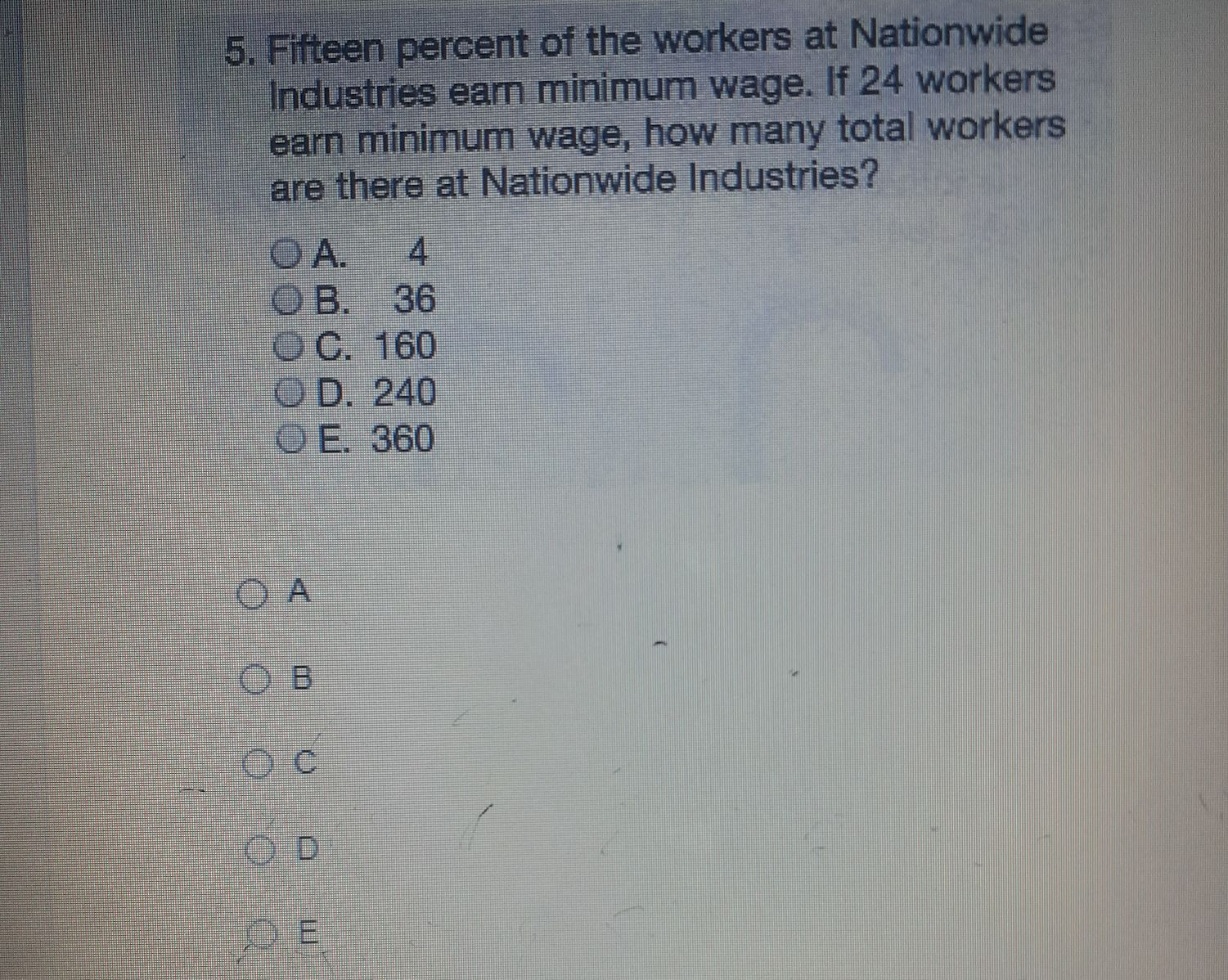### Still have math questions?

Arithmetic
Question5. Fifteen percent of the workers at Nationwide Industries earn minimum wage. If $$24$$ workers earn minimum wage, how many total workers are there at Nationwide Industries?

A. $$4$$ B. $$36$$ C. $$160$$ D. $$240$$ E. $$360$$